5 Derivation of Basic Equations

We are looking for two inter-related functions Y and K. For mathematical reasons it is clear that we need two coupled, linear independent equations for the determination. Linearly independent does not mean that the determination must be linear equations. Linearly independent states, that there is no way to derive one equation from the other by simple (linear) arithmetic operations. But experience shows that a linear approach of defining equations is often already correct, or at least close to it. You might find the desired equations of motion of the macro economy to develop on the Lagrangeformalism directly. However, this approach is mathematically neither trivial nor intuitive. We shall come back to that later. We will as first develop the equations of motion in an intuitive way, by balancing them correctly, and thus bring them about. We come in, because the two functions must be sought in mutual dependency: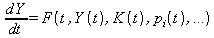(5.1 a)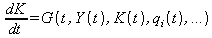(5.1 b)

The differential functions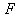and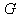ad hoc, however, are unknown. What is certain is that they must be dependent on time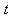and of the other factors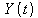and. In addition there are other parameters that can possibly time-dependent functions and which, for example, are interest percentages or other parameters.